# What does this package do?

The `countcolors` package finds pixels by color range in an image. It started as a collaboration between Sarah Hooper, Sybill Amelon, and me (Hannah Weller), in order to quantify the area of white-nose syndrome infection on bat wings. In general, it’s meant to substitute for manual region-of-interest selection, which can be time-consuming and inconsistent.

# How it works

`countcolors` deals primarily with RGB color space. You’re probably familiar with the fact that digital images are stored with three color layers: red, green, and blue. We can think of these like the dimensions of a three-dimensional color space1, where a color is defined by its red, green, and blue coordinates. Each of these axes ranges from 0 to 255 (or 0 to 1, if we’re dealing with unit vectors). So pure red, for example, would have a red value of 1, a green value of 0, and a blue value of 0. Magenta, which is an equal mix of red and blue, would have an RGB triplet of [1, 0, 1].

``## Loading required package: scatterplot3d``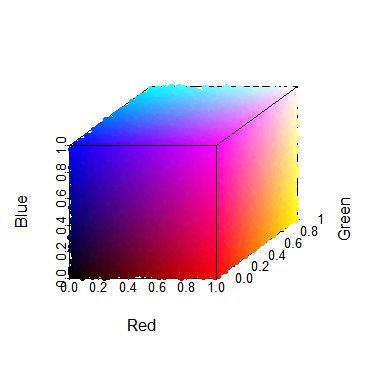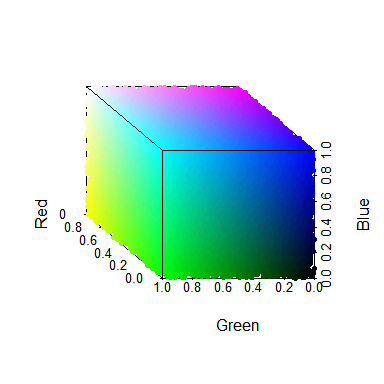To search for pixels of a certain color, `countcolors` draws a set of boundaries around a region of color space, and counts all the pixels in the image that fall within those boundaries. There are two ways of defining the boundaries: either you can set upper and lower limits for each color channel, drawing a bounding ‘box’ around a region of color space, or you can pick a color center and a radius to search around it, which draws a ‘sphere’ in color space.

## Rectangular ranges

If we wanted to define ranges for magenta pixels, we would look for pixels near the RGB value of [1, 0, 1]. To expand this range a little, we could define the following conditions:

• Red: 0.7 to 1.0
• Green: 0 to 0.3
• Blue: 0.7 to 1.0

Then we search for pixels whose colors satisfy all three conditions. In `countcolors`, you do this by specifying a lower and an upper set of RGB triplets: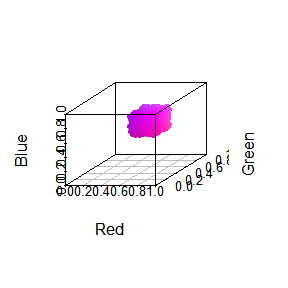This is why it’s referred to as a ‘rectangular’ range – it draws a box around a region of color space.

## Spherical ranges

Sometimes, rather than boundaries, you want to specify a particular color and a search radius around that color. `countcolors` uses this to draw a sphere in color space centered around a single color, whose size depends on the radius. Say we’re looking at a mossy green color, with an RGB triplet of [0.4, 0.7, 0.4]. If we set the radius very small – say, 5% of maximum color distance – we only get a few pixels back: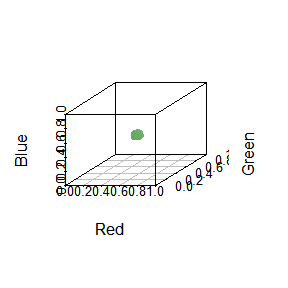But if we increase that radius to 25%, we get a much bigger search space and many more pixels back: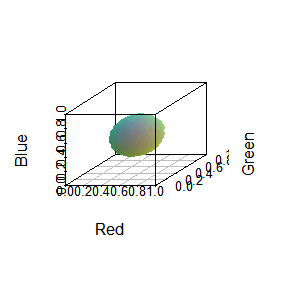All `countcolors` does is search for pixels within a color range specified by the user, count them, and tell you how many there are and where they are in the image. It also comes with a couple of diagnostics to check that you’re picking the right color range.

1: Of course, there are many color spaces besides RGB, such as HSV (probably familiar), CMYK (maybe familiar), and CIELab (probably only familiar if you’ve worked with color spaces before, in which case you definitely don’t need my explanation). RGB is actually considered quite a poor representation of how human beings perceive color, but it works just fine for quantifying color proportions. A full discussion of color spaces is well beyond the scope of this introduction, but if you want to know more, I recommend Bruce Lindbloom’s website.

# Example of basic workflow

To see how this works in practice, let’s look at an aerial photograph of Norway from NASA: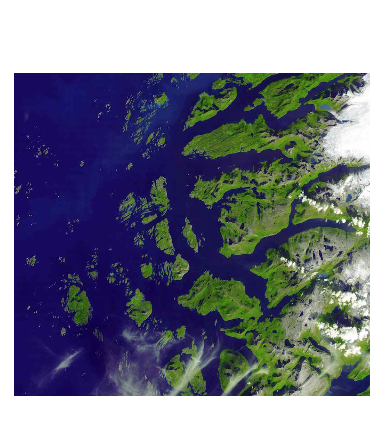We’re mostly dealing with green, dark blue, and white in this image. If we plot them in color space, we can see where those colors are:

``````# Note we're using the `plotPixels` function from the related colordistance
# package
colordistance::plotPixels("norway.jpg", lower = NULL, upper = NULL, n = 5000)``````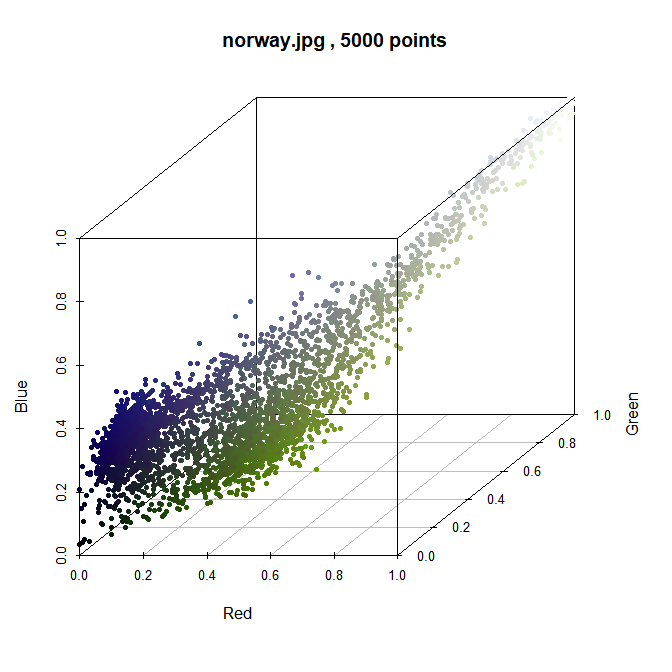The pixels mostly group into blue, white, or green clusters, as expected, but of course they aren’t all perfectly centered. For this example, let’s say we care about the amount of plant cover on the coastline, so we want to calculate the percentage of green in the image. To do that, we’ll define a range in which to search for green pixels, calculate the percent cover, and then check whether we actually chose to the right parameters by changing all the targeted pixels to a different color.

## Finding the right color range

The success of any of the functions in `countcolors` relies on providing them with the right color range(s).To find the color range, there are a few things you can try, including:

1. Open the image in ImageJ, Preview, Photoshop, or another image viewer and finding the exact RGB triplets of a few pixels in the area of interest using a color picker.

2. Plot the pixels in color space to look for approximate natural boundaries, like above.

3. Use k-means clustering to find natural groupings of colors, together with exact centers2.

That last one is obviously a little more complicated, but we can actually use `colordistance` to do it in one line:

``````# Find K-means clusters
kmeans.clusters <- colordistance::getKMeanColors("norway.jpg", n = 3, plotting = FALSE)
colordistance::extractClusters(kmeans.clusters)``````

To plot the clusters in an interactive plot, you can use `colordistance::plotClusters`, but for now we’ll just look at the clusters themselves. The green one is the third row: [0.34, 0.45, 0.24]. We’ll use that as our guideline and try a couple of different radii (for spherical ranges) and boundaries (for rectangular ranges). In `countcolors`, ranges are specified using RGB triplets, which are just vectors of length 3 assumed to be in R-G-B order.

``````center.spherical <- c(0.24, 0.45, 0.24) # Center color for spherical range

lower.rectangular <- c(0.2, 0.35, 0.2) # Lower limit for each of the three color channels
upper.rectangular <- c(0.3, 0.55, 0.3) # Upper limit for each of the three color channels``````

2: It’s tempting to just use the output of the k-means clustering, but this usually doesn’t work as well as you might think. Because of the way the algorithm works, it tends to either group colors together or artificially break them up into a large number of clusters. In this case, it works because the green, blue, and white are sufficiently far apart that pixels from one don’t get assigned to the other color’s cluster, but in more complicated images this is very common. K-means is better as a guideline.

## Counting the pixels

Now that we have color ranges to try out, we can actually use the functions. The main function of `countcolors` is, unsurprisingly, `countColors`, which calls on the other functions of the package to do three things:

1. Find the locations of pixels within a target range or ranges;
2. Find the proportion of the image (optionally ignoring the background) covered by those pixels;
3. Provide a version of the image with those pixels masked out in order to check whether the function worked.

First, we’ll walk through what the function does step-by-step, using the functions that `countColors` actually calls on. Then we’ll see how use `countColors` to do all of that under the roof of one function.

``````# Read the image into the R environment

# Find all the pixels within a 10% radius
norway.spherical <- countcolors::sphericalRange(norway, center = center.spherical, radius = 0.1, color.pixels = FALSE, plotting = FALSE); names(norway.spherical)``````
``##  "pixel.idx"    "pixel.count"  "img.fraction" "original.img"``
``norway.spherical\$img.fraction``
``##  0.1372811``

`sphericalRange` accepts a color center and a radius to define a color search space, and returns a list including row and column indices of the pixels within the range (`pixel.idx`), the number of pixels within that range (`pixel.count`), the fraction of the image covered by that amount (`img.fraction`), and the original RGB array.

Above, we started with a fairly conservative 10% radius around the color, which apparently covers about 13.7% of the image. It’s hard to tell if that’s the right proportion without seeing it, so the next step is to mask out the pixels within that range using `changePixelColor` to see if we missed anything:

``countcolors::changePixelColor(norway, norway.spherical\$pixel.idx, target.color="magenta")``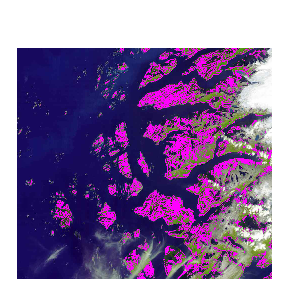We got most of the visible green, but we’re clearly missing an awful lot here. One option is to nudge the center color around, but first we’ll try increasing the radius. We’ll also set `plotting = TRUE` in the `sphericalRange` function, which calls on `changePixelColor` to generate the masked image:

``````# Find all the pixels within a 17% radius
norway.spherical <- countcolors::sphericalRange(norway,
center = center.spherical, radius = 0.15,
color.pixels = FALSE, plotting = TRUE,
target.color = "magenta"); norway.spherical\$img.fraction``````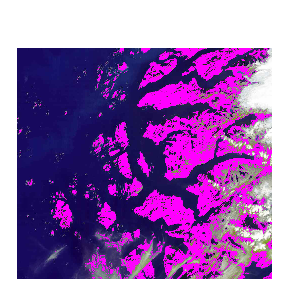``##  0.260676``

That’s more like it! It looks like the green is masked out by magenta, but not the ocean, the clouds, or the mountaintops. Of course, the 15% radius was arrived at cooking-show style after I tested a few different radii that were too high or too low, and most images will require some experimentation.

Using a rectangular range is a very similar procedure:

``````# Trying with our original color ranges
norway.rectangular <- countcolors::rectangularRange(norway,
upper = upper.rectangular, lower = lower.rectangular,
target.color = "yellow")``````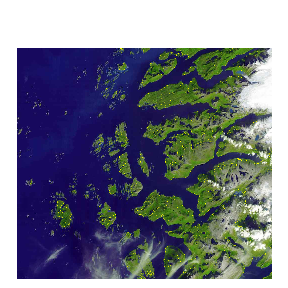``````# Trying with our cooking show values
norway.rectangular <- countcolors::rectangularRange(norway,
upper = c(0.55, 0.75, 0.4), lower = c(0.1, 0.25, 0),
target.color = "yellow"); norway.rectangular\$img.fraction``````